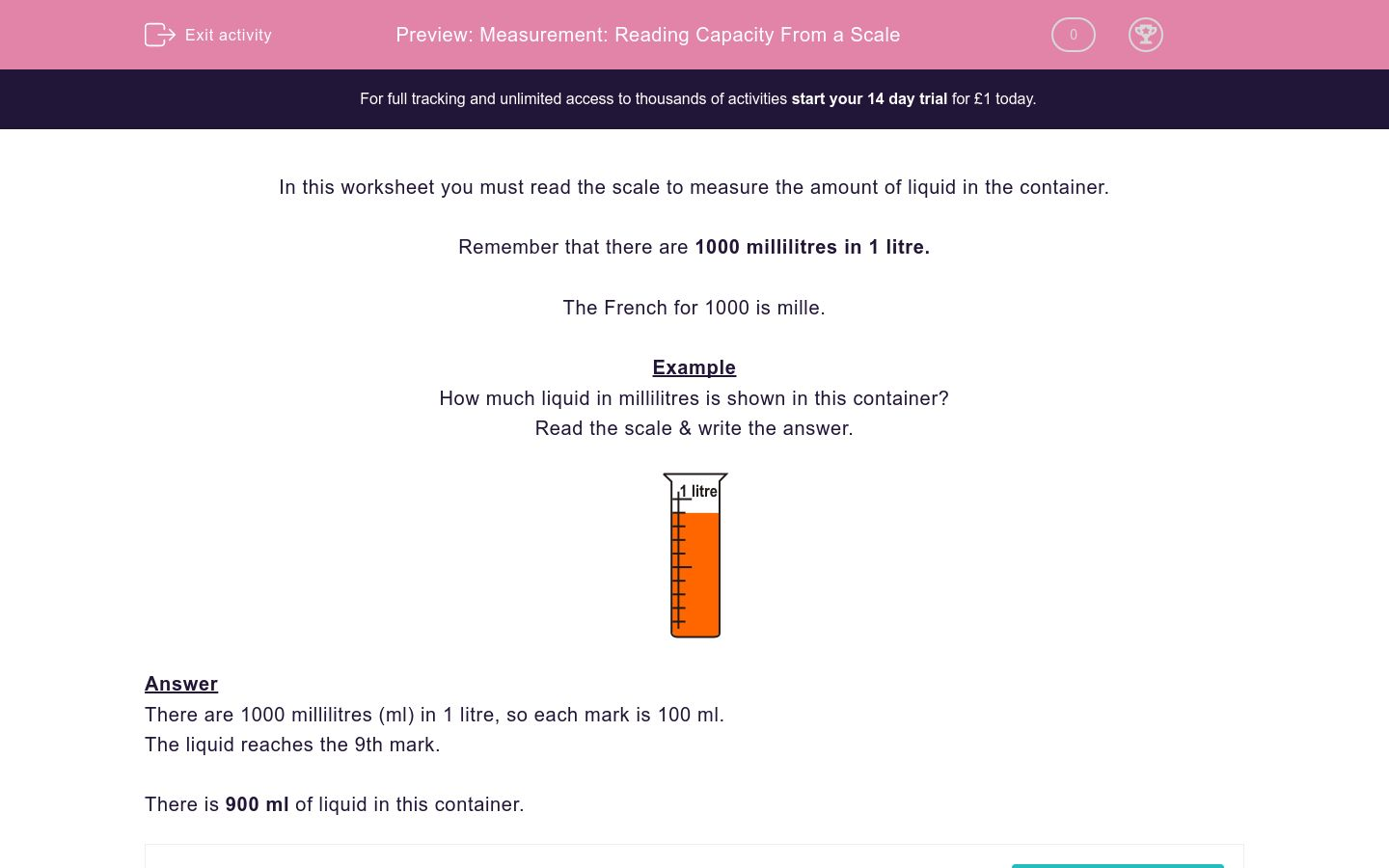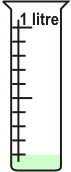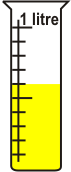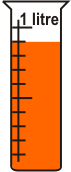# Measurement: Reading Capacity From a Scale

In this worksheet, students measure the amount of liquid in a container by reading a scale.Key stage:  KS 2

Curriculum topic:   Maths and Numerical Reasoning

Difficulty level:### QUESTION 1 of 10

In this worksheet you must read the scale to measure the amount of liquid in the container.

Remember that there are 1000 millilitres in 1 litre.

The French for 1000 is mille.

Example

How much liquid in millilitres is shown in this container?There are 1000 millilitres (ml) in 1 litre, so each mark is 100 ml.

The liquid reaches the 9th mark.

There is 900 ml of liquid in this container.

How much liquid in millilitres is shown in this container?

(just write the number)How much liquid in millilitres is shown in this container?

(just write the number)How much liquid in millilitres is shown in this container?

(just write the number)How much liquid in millilitres is shown in this container?

(just write the number)How much liquid in millilitres is shown in this container?

(just write the number)How much liquid in millilitres is shown in this container?

(just write the number)How much liquid in millilitres is shown in this container?

(just write the number)How much liquid in millilitres is shown in this container?

(just write the number)How much liquid in millilitres is shown in this container?

(just write the number)How much liquid in millilitres is shown in this container?

(just write the number)• Question 1

How much liquid in millilitres is shown in this container?

(just write the number)100
EDDIE SAYS
1st mark.
• Question 2

How much liquid in millilitres is shown in this container?

(just write the number)700
EDDIE SAYS
7th mark.
• Question 3

How much liquid in millilitres is shown in this container?

(just write the number)600
EDDIE SAYS
6th mark.
• Question 4

How much liquid in millilitres is shown in this container?

(just write the number)300
EDDIE SAYS
3rd mark.
• Question 5

How much liquid in millilitres is shown in this container?

(just write the number)200
EDDIE SAYS
2nd mark.
• Question 6

How much liquid in millilitres is shown in this container?

(just write the number)800
EDDIE SAYS
8th mark.
• Question 7

How much liquid in millilitres is shown in this container?

(just write the number)400
EDDIE SAYS
4th mark.
• Question 8

How much liquid in millilitres is shown in this container?

(just write the number)1000
EDDIE SAYS
10th mark.
• Question 9

How much liquid in millilitres is shown in this container?

(just write the number)500
EDDIE SAYS
1st mark.
• Question 10

How much liquid in millilitres is shown in this container?

(just write the number)900
EDDIE SAYS
9th mark.
---- OR ----